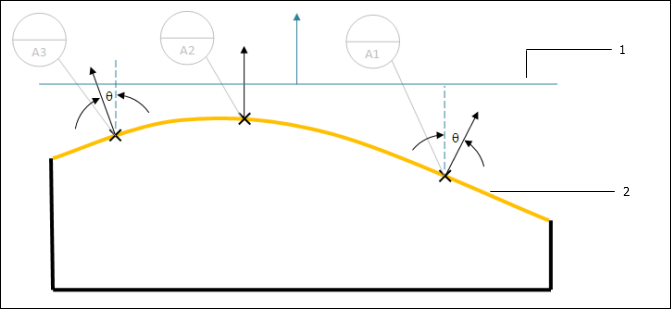The Specified Targets Do Not Define a Stable Plane
Ideally the surface normal vector at each datum target is nearly parallel to the normal of the related datum plane. As that angle approaches 90 degrees, the datum plane becomes unstable and has poor repeatability. The TGT_NormAngle value (defined in the system application options) specifies the maximum allowable angle between the surface normal vector at the target points and the normal vector of the datum plane. This message is displayed when the angle exceeds that value.
The example pictured below shows part with 3 datum target points and the surface normal vector at each point. The blue plane represents the datum plane that is established by the datum target points.1. Datum plane
2. Feature
In order to address this problem, you should modify the targets in the design model such that the surface normals at the targets are more nearly parallel to the normal vector of the datum plane.